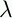September 22, 1999 Perl and the Lambda Calculus Slide #31

```        Y =f.(x.(f (x x))x.(f (x x)))
```
• Actually many others are possible, but this is the simplest

• Claim: (Y f) = (f (Y f))

• Let's see:

```        (Y f)
```
```        (f.(x.(f (x x))x.(f (x x))) f)
```
```        (x.(f (x x))x.(f (x x)))
```
```        (f (x.(f (x x))x.(f (x x))))
```
• Why look, that's exactly (f (Y f)).

• This is f making a recursive call

• If another recursive call is needed the new (Y f) will generate one

• Etc.

 NextCopyright © 1999 M-J. Dominus